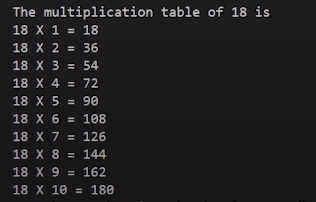### C Program to Generate Multiplication Table of a Given Number

In this tutorial, we will write a program for multiplication of tables of a given number in C language. An example program is shown below

``````#include<stdio.h>

int main(){
int num;
// Take the number as an input from the user
printf("Enter the value of number whose multiplication table is to be printed\n");
scanf("%d", &num);
printf("The multiplication table of %d is\n", num);
for (int i = 0; i < 10; i++)
{
printf("%d X %d = %d\n",num, i+1, (i+1)*num);
}

return 0;
}
``````

Code Snippet 1: Multiplication of Table of a Given Number

#### Explanation

As shown in a code snippet 1,

1. We have declared an integer variable “num” which will be used to store user input
2. The “printf” function is used to print “Enter the value of number whose multiplication table is to be printed” at the run time and “/n” will break the line
3. The “scanf” function is used to get input from user; the “%d” refer to an integer and “num” is the variable in which the user input will be stored
4. The “printf” function is used to print “The multiplication table of %d is”, the value of the variable “num” will be printed at the place of “%d”
5. The “for” loop is used to iterate for the given number of times. The “printf” function inside “for” loop is used to print the multiplication table. Every times “for” loops iterates it work print the given number, value of (i+1), and value of (i+1) will be multiplied by the given number. For example if the user input the number 4 then the output will be like:

4 X 1 = 4

4 X 2 = 8

And so on till the value of “i” reaches the value 10. There is another logic to iterate the for loop without adding the value “1” in the variable “i” which is shown below

``````for (int i = 1; i <= 10; i++)
{
printf("%d X %d = %d\n",num, i, i*num);
}
``````

Code Snippet 2: Another way to print table

As shown in the code snippet 2,

1. In this for loop the value of the variable “i” is set to “1” and the loop condition is set to “i <= 10”; due to which we don’t need to add “1” in the value of the variable “i”

The output of the following program is shown belowFigure 1: Program Output

## You must be logged in to post a comment!

### Comments(1)subrata01 3 months ago
`sir can not find the link of hand written notes..`

#### Resources

No downloadable resources for this video. If you think you need anything, please post it in the QnA!

#### Course Announcements

Any Course related announcements will be posted here